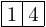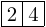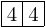Queries for Semistandard tableaux: search statistic / browse statistics / browse maps from / browse maps to

# Definition & Example

• A semistandard (Young) tableau of a partition $\lambda \vdash n$ is a map from the cells of the Young diagram of $\lambda$ to $\{1,2,\dots,r\}$ for some $r$, such that rows are weakly and columns are strictly increasing.

• The set of semistandard tableaux of shape $\lambda \vdash n$ with maximal possible entry $r$ is denoted by $\mathcal{SSYT}_{\lambda,r}$, and

$$\mathcal{SSYT}_{n,r} = \bigcup_{\lambda \vdash n}\mathcal{SSYT}_{\lambda,r}$$
denotes the set of semistandard tableaux of size $n$.

 the 7 Semistandard tableaux of size (2, 4)[[1,4]] [[2,4]] [[3,4]] [[4,4]] [,] [,] [,]

• Semistandard tableaux are graphically represented by filling the cells of the Young diagram in English notation.

# Properties

• The number of semistandard tableaux of a partition $\lambda$ with all entries in $\{1,\ldots,r\}$ is given by the hook-content formula
$$\big|\mathcal{SST}_\lambda\big| = \prod_{(i,j)}\frac{r+j-i}{hook(i,j)},$$
where the product ranges over all boxes $(i,j)$ in the Young diagram of $\lambda$, and where $hook(i,j)$ is the hook length of the box$(i,j)$ in $\lambda$.

# Remarks

• Semistandard tableaux with maximal entry at most $M$ are in bijection with Gelfand-Tsetlin patterns with length of first row equal to $M$. This yields a natural surjection from Gelfand-Tsetlin patterns to semistandard Young tableaux.

• Skew Tableaux come from taking the set difference of two partitions of different sizes. If the resulting boxes are filled with numbers such that the columns are increasing and the rows are weakly increasing, then it is called a semistandard skew tableaux.

• The number of semistandard tableaux of shape $\lambda$ and content $\mu$ is equal to the Kostka number $K_{\lambda\mu}$. The hook length formula is a special case when $\mu = (1,1,1,...,1)$, since that corresponds to a standard tableau. However, there is no general formula for the Kostka numbers.

# Technical information for database usage

• A semistandard tableau is uniquely represented as a list of lists giving the fillings of the cells row by row.
• Semistandard tableaux are are bigraded by two integers $n$ and $m$, where $n$ is the size of the underlying partition and $m$ is the maximal occurring entry.
• The database contains all semistandard tableaux such that $n+m$ is at most 8.

If you want to edit this wiki page, you can download the raw markdown and send your new version to info@findstat.org